International
Tables for
Crystallography
Volume D
Physical properties of crystals
Edited by A. Authier

International Tables for Crystallography (2013). Vol. D, ch. 1.11, pp. 277-278

Section 1.11.6.3. Hidden internal symmetry of the dipole–quadrupole tensors in resonant atomic factors

V. E. Dmitrienko,a* A. Kirfelb and E. N. Ovchinnikovac

aA. V. Shubnikov Institute of Crystallography, Leninsky pr. 59, Moscow 119333, Russia,bSteinmann Institut der Universität Bonn, Poppelsdorfer Schloss, Bonn, D-53115, Germany, and cFaculty of Physics, M. V. Lomonosov Moscow State University, Leninskie Gory, Moscow 119991, Russia
Correspondence e-mail:  dmitrien@crys.ras.ru

1.11.6.3. Hidden internal symmetry of the dipole–quadrupole tensors in resonant atomic factors

| top | pdf |

It is fairly obvious from expressions (1.11.6.3)and (1.11.6.16)that in the non-magnetic case the symmetric and antisymmetric third-rank tensors,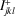and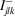, which describe the dipole–quadrupole contribution to the X-ray scattering factor, are not independent: the antisymmetric part, which is also responsible for optical-activity effects, can be expressed via the symmetric part (but not vice versa). Indeed, both of them can be described by a symmetric third-rank tensor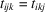resulting from the second-order Born approximation (1.11.6.3),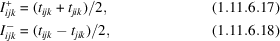where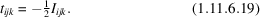From equation (1.11.6.17), one can infer that the symmetry restrictions for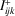andare the same. Then it can be seen thatcan be expressed via.

For any symmetry,andhave the same number of independent elements (with a maximum 18 for site symmetry 1). Thus, one can reverse equation (1.11.6.17)and expressdirectly in terms of: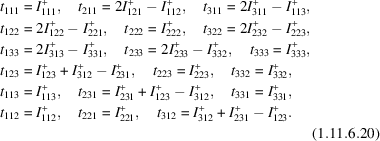Using equations (1.11.6.18)and (1.11.6.20), one can express all nine elements ofthrough: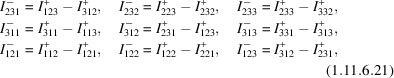according to which the antisymmetric part of the dipole–quadrupole term is a linear function of the symmetric one [however, not vice versa: equations (1.11.6.21)cannot be reversed].

Note that the equations (1.11.6.21)impose an additional restriction on, which applies to all atomic site symmetries:This is, in fact, a well known result: the pseudo-scalar part ofvanishes in the dipole–quadrupole approximation used in equation (1.11.6.3). Thus, for point symmetry 1,has only eight independent elements rather than nine. This additional restriction works in all cases of higher symmetries provided the pseudo-scalar part is allowed by the symmetry (i.e. point groups 2, 3, 4, 6, 222, 32, 422, 622, 23 and 432). All other symmetry restrictions onarise automatically from equation (1.11.6.21)taking into account the symmetry of[symmetry limitations onandfor all crystallographic point groups can be found in Sirotin & Shaskolskaya (1982) and Nye (1985)].

Let us consider two examples, ZnO and anatase, TiO2, where the dipole–dipole contributions to forbidden reflections vanish, whereas both the symmetric and antisymmetric dipole-quadrupole terms are in principal allowed. In these crystals, the dipole–quadrupole terms have been measured by Goulon et al. (2007) and Kokubun et al. (2010).

In ZnO, crystallizing in the wurtzite structure, the 3m symmetry of the atomic positions imposes the following restrictions on: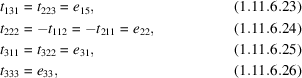where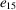,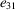,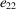,are energy-dependent complex tensor elements [keeping the notations by Sirotin & Shaskolskaya (1982), the x axis is normal to the mirror plane, the y axis is normal to the glide plane and the z axis corresponds to the c axis of ZnO]. If we suppose these restrictions for Zn at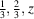, then for the other Zn at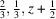, which is related to the first site by the glide plane, there is the following set of elements: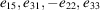. Therefore, the structure factors of the glide-plane forbidden reflections are proportional to.

For the symmetric and antisymmetric parts one obtains from equations (1.11.6.17)and (1.11.6.18)the non-zero components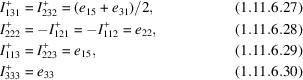and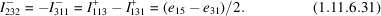Physically, we can expect thatbecause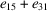survives even for tetrahedral symmetry, whereas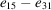is non-zero owing to a deviation from tetrahedral symmetry; in ZnO, the local coordinations of the Zn positions are only approximately tetrahedral.

In the anatase structure of TiO2, thesymmetry of the atomic positions imposes restrictions on the tensors[keeping the notations of Sirotin & Shaskolskaia (1982): the x and y axes are normal to the mirror planes, and the z axis is parallel to the c axis]:whereandare energy-dependent complex parameters. If we apply these restrictions to the Ti atoms atand, then for the other two inversion-related Ti atoms at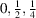and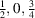(centre), the parameters are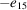and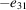.

For the symmetric and antisymmetric parts one obtains as non-vanishing componentsand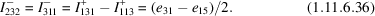It is important to note that the symmetric partof the atomic factor can be affected by a contribution from thermal-motion-induced dipole–dipole terms. The latter terms are tensors of rank 3 proportional to the spatial derivatives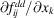, which take the same tensor form asbut are not related toby equations (1.11.6.21). In ZnO, which was studied in detail by Collins et al. (2003), the thermal-motion-induced contribution is rather significant, while for anatase the situation is less clear.

References

Collins, S. P., Laundy, D., Dmitrienko, V., Mannix, D. & Thompson, P. (2003). Temperature-dependent forbidden resonant X-ray scattering in zinc oxide. Phys. Rev. B, 68, 064110.
Goulon, J., Jaouen, N., Rogalev, A., Wilhelm, F., Goulon-Ginet, C., Brouder, C., Joly, Y., Ovchinnikova, E. N. & Dmitrienko, V. E. (2007). Vector part of optical activity probed with X-rays in hexagonal ZnO. J. Phys. Condens. Matter, 19, 156201.
Kokubun, J., Sawai, H., Uehara, M., Momozawa, N., Ishida, K., Kirfel, A., Vedrinskii, R. V., Novikovskii, N., Novakovich, A. A. & Dmitrienko, V. E. (2010). Pure dipole–quadrupole resonant scattering induced by the p–d hybridization of atomic orbitals in anatase TiO2. Phys. Rev. B, 82, 205206.
Nye, J. F. (1985). Physical Properties of Crystals: Their Representation by Tensors and Matrices. Oxford University Press.
Sirotin, Y. & Shaskolskaya, M. P. (1982). Fundamentals of Crystal Physics. Moscow: Mir.